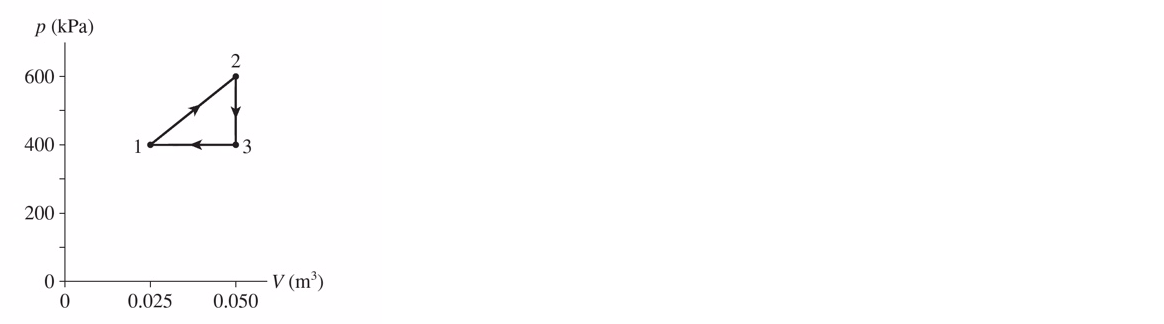# Problem: An engine uses 1.4 mol of a monoatomic ideal gas undergoing the cycle shown in the following figure. a) Determine T1, T2, and T3.b) Make a table showing ΔU, W, and Q for each step of the cycle. c) What is the engine's thermal efficiency?

###### FREE Expert Solution
86% (178 ratings)
###### Problem Details

An engine uses 1.4 mol of a monoatomic ideal gas undergoing the cycle shown in the following figure.

a) Determine T1, T2, and T3.

b) Make a table showing ΔU, W, and Q for each step of the cycle.

c) What is the engine's thermal efficiency?Frequently Asked Questions

What scientific concept do you need to know in order to solve this problem?

Our tutors have indicated that to solve this problem you will need to apply the Heat Engines & PV Diagrams concept. You can view video lessons to learn Heat Engines & PV Diagrams. Or if you need more Heat Engines & PV Diagrams practice, you can also practice Heat Engines & PV Diagrams practice problems.